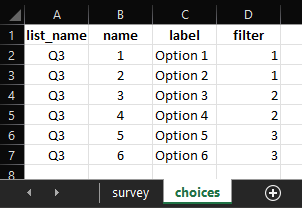# How to remove choices of an answer depending on previous questions (displaying specific choices as required by the context in a later question when selecting certain choices in the previous question)

Hi,

I have 3 questions, the first and second questions are likert scales (1 to 5).
The answers (choices) of third question is depending on the answers of 1 and 2nd questions, If answers of Q1 and Q2 are the same, then choices 1 and 2 of Q3 will be appearing, if answers of Q1 is greater than Q2, the choices 3 and 4 of Q3 will be appearing, and if answers of Q1 is less than Q2, the choices 5 and 6 of Q3 will be appearing. What methodology should I use to do that. Thanks for your help.

@botani, you could do the same as outlined in the image shared below:

#### In the choices tab of your XLSForm:#### Reference XLSForm:

Range Question Type.xlsx (10.8 KB)

1 Like

Hi again,